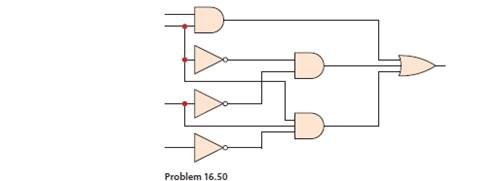Chapter 16, Problem 50P

Chapter
Section
Textbook Problem

Using Table 16.1, identify the components of the logic system shown in the figure.To determine

Identify the components of the logic diagram that are used in Figure Problem 16.50 in the textbook.

Explanation

Calculation:

Sketch the given in Figure 1 as follows,

Refer to Table 16.1 in the textbook, it shows the engineering symbols for various components. The given figure consists of logic gates components, and shows the logic gates symbols in second section.

The name of the components which are used in the circuit is given below with refer to that Table 16.1.

AND gate:

Still sussing out bartleby?

Check out a sample textbook solution.

See a sample solution

The Solution to Your Study Problems

Bartleby provides explanations to thousands of textbook problems written by our experts, many with advanced degrees!

Get Started

What does the term 3 Vs refer to?

Database Systems: Design, Implementation, & Management

Describe the difference between wheel truing and dressing.

Precision Machining Technology (MindTap Course List)

List steps to add a computer or mobile device to a wireless Network.

Enhanced Discovering Computers 2017 (Shelly Cashman Series) (MindTap Course List)